Maths-
General
Easy

Question

# If a, b, c are in A.P then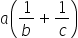,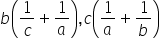are in

## A.P.    G.P.    H.P.    A.G.PHint:

## The correct answer is: A.P.

### Given : a, b , c are in AP.We know that in AP2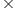second term = first term + third term2b = a + cNow, (1)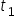==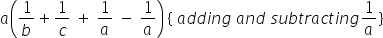Let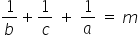(2) Term2 (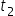) =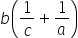Term2 () =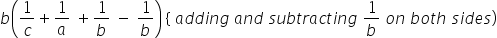Term2 () =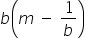Term2 () =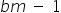Similarly,(3) Term3 (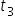) =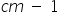Let,,be in APWe can check if they satisfy the condition 2b = a + c2=+Substituting the values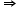2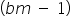=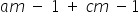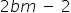=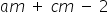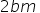=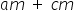Taking m common and cancelling out from both sides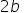=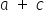Thus,  a, b, c are in A.P then,are in A.P.#### With Turito Foundation.#### Get an Expert Advice From Turito.